# INTRODUCTION¶

There are several ways of making money in the stock market; here we explore a few of them using techniques from data science and finance. We explore alpha generation from the perspectives of:

• leveraged finance (ETF decay) and similar tricks of the trade
• digital signal processing
• portfolio construction using MPT (Modern Portfolio Theory)
• bayesian Natural Language Processing using Twitter data, attempting to perform sentiment analysis

## Initial Question¶

Thus our initial question is: Which of the above methods will generate alpha, and of course what are the caveats.

## Motivation¶

The motivation of this project is to apply knowledge from 209 to finance. The motivation is to make money. We not only want to just maximize returns, but also minimize volitility. You will see through the statistics we employ, that we focused on these two ideas. That is our motivation.

In terms of related work. Many of our funcitons are derived from other works (like the butterworth filter). We have sited them in our .py files. In addition we have used what we learned on bayesian tomatoes quite extensively. Most of the other work we have done, comes from our own experience.

## Data Munging¶

We colleted the initial data from Quandl, and have included it as files in the .zip. In addition, we collected twitter data. That process is descibed below.

## Exploratory Analysis¶

Grappling with timeseries data is essential to our task of stock price prediction, since a stock traces out a series of datapoints with regard to time. Fortunately, pandas includes an API for time-series manipulations. In the following, we introduce some exploratory statistics and methodology and begin to analyze stock data from a returns perspective.

The pandas time-series API includes:

• 1.) Date ranges from files and from scratch
• 2.) Shift, resample, and filter manipulations
• 3.) Field accessors
• 4.) Plotting
• 5.) Time zones (localization and conversion)
• 6.) Dual representations (point-in-time vs interval)

Let's import some code. From datetime, we need

• datetime
• date
• time

From pandas, let's get the timeseries tools:

• Series
• DataFrame
• Panel

• matplotlib
• sys
In :
# Code to allow inline plotting, derived from psets

import warnings
warnings.filterwarnings('ignore')
from helperfile import *
plt.rc('figure', figsize=(10, 6))

%matplotlib inline

import json

import requests
import pandas as pd
import numpy as np
import matplotlib.pyplot as plt

pd.set_option('display.width', 500)
pd.set_option('display.max_columns', 30)

# set some nicer defaults for matplotlib
from matplotlib import rcParams

#these colors come from colorbrewer2.org. Each is an RGB triplet
dark2_colors = [(0.10588235294117647, 0.6196078431372549, 0.4666666666666667),
(0.8509803921568627, 0.37254901960784315, 0.00784313725490196),
(0.4588235294117647, 0.4392156862745098, 0.7019607843137254),
(0.9058823529411765, 0.1607843137254902, 0.5411764705882353),
(0.4, 0.6509803921568628, 0.11764705882352941),
(0.9019607843137255, 0.6705882352941176, 0.00784313725490196),
(0.6509803921568628, 0.4627450980392157, 0.11372549019607843),
(0.4, 0.4, 0.4)]

rcParams['figure.figsize'] = (10, 6)
rcParams['figure.dpi'] = 150
rcParams['axes.color_cycle'] = dark2_colors
rcParams['lines.linewidth'] = 2
rcParams['axes.grid'] = False
rcParams['axes.facecolor'] = 'white'
rcParams['font.size'] = 14
rcParams['patch.edgecolor'] = 'none'

def remove_border(axes=None, top=False, right=False, left=True, bottom=True):
"""
Minimize chartjunk by stripping out unnecessary plot borders and axis ticks

The top/right/left/bottom keywords toggle whether the corresponding plot border is drawn
"""
ax = axes or plt.gca()
ax.spines['top'].set_visible(top)
ax.spines['right'].set_visible(right)
ax.spines['left'].set_visible(left)
ax.spines['bottom'].set_visible(bottom)

#turn off all ticks
ax.yaxis.set_ticks_position('none')
ax.xaxis.set_ticks_position('none')

#now re-enable visibles
if top:
ax.xaxis.tick_top()
if bottom:
ax.xaxis.tick_bottom()
if left:
ax.yaxis.tick_left()
if right:
ax.yaxis.tick_right()


Let's sample some data from the SPX.csv data file, sourced from Yahoo Finance S&P 500.

In :
with open('SPX.csv', 'r') as ff:

DATE,OPEN,HIGH,LOW,CLOSE,VOLUME,ADJ

2013-09-13,169.13,169.46,168.74,169.33,72459700,169.33



We can use lists or dicts for parsing dates.

In :
data = pd.read_csv('SPX.csv',parse_dates={'Timestamp': ['DATE']},index_col='Timestamp')
data

Out:
<class 'pandas.core.frame.DataFrame'>
DatetimeIndex: 5195 entries, 2013-09-13 00:00:00 to 1993-01-29 00:00:00
Data columns (total 6 columns):
OPEN      5195  non-null values
HIGH      5195  non-null values
LOW       5195  non-null values
CLOSE     5195  non-null values
VOLUME    5195  non-null values
dtypes: float64(5), int64(1)


Let's take a look at some of these more recent values:

In :
history = data.ix[:, ['OPEN', 'VOLUME']]

Out:
OPEN VOLUME
Timestamp
2013-09-13 169.13 72459700
2013-09-12 169.34 83098200
2013-09-11 168.64 94272700
2013-09-10 168.64 102432800
2013-09-09 166.45 83392900

Compute a VWAP (Volume Weighted Avereage Price, a weighted avereage of price with volume as the weights, often used as an anchoring quantity for algorithmic traders) using resample.

In :
volume = history.VOLUME.resample('1w', how='sum') # weekly vwap
value = history.prod(axis=1).resample('1w', how='sum')
vwap = value / volume
print vwap

Timestamp
1993-01-31    43.970000
1993-02-07    44.554778
1993-02-14    44.845201
1993-02-21    44.021129
1993-02-28    43.816993
1993-03-07    44.786620
1993-03-14    45.346889
1993-03-21    45.133627
1993-03-28    44.827772
1993-04-04    45.121409
1993-04-11    44.453826
1993-04-18    44.844166
1993-04-25    44.560004
1993-05-02    43.723734
1993-05-09    44.391409
...
2013-06-09    163.200245
2013-06-16    163.581478
2013-06-23    162.626114
2013-06-30    159.262948
2013-07-07    161.399900
2013-07-14    165.689620
2013-07-21    168.265996
2013-07-28    169.031879
2013-08-04    169.393906
2013-08-11    169.890286
2013-08-18    167.850913
2013-08-25    165.400218
2013-09-01    164.397174
2013-09-08    165.588047
2013-09-15    168.435810
Freq: W-SUN, Length: 1077, dtype: float64


We can conveniently index financial time series data as follows.

In :
vwap.ix['1993-01-31':'2013-09-15']

Out:
Timestamp
1993-01-31    43.970000
1993-02-07    44.554778
1993-02-14    44.845201
1993-02-21    44.021129
1993-02-28    43.816993
1993-03-07    44.786620
1993-03-14    45.346889
1993-03-21    45.133627
1993-03-28    44.827772
1993-04-04    45.121409
1993-04-11    44.453826
1993-04-18    44.844166
1993-04-25    44.560004
1993-05-02    43.723734
1993-05-09    44.391409
...
2013-06-09    163.200245
2013-06-16    163.581478
2013-06-23    162.626114
2013-06-30    159.262948
2013-07-07    161.399900
2013-07-14    165.689620
2013-07-21    168.265996
2013-07-28    169.031879
2013-08-04    169.393906
2013-08-11    169.890286
2013-08-18    167.850913
2013-08-25    165.400218
2013-09-01    164.397174
2013-09-08    165.588047
2013-09-15    168.435810
Freq: W-SUN, Length: 1077, dtype: float64
In :
selection = vwap.between_time('1993-01-31', '2013-09-15')
vol = pd.Series(volume.between_time('1993-01-31', '2013-09-15'),dtype=float)
# attempt to fill possible missing data
vol = vol.fillna(0.)
# now for visualization, plot the volume weighted avereage price, along with volume, with respect to time.
vol.plot(style='y')
filled.plot(secondary_y=True, style='r')
remove_border()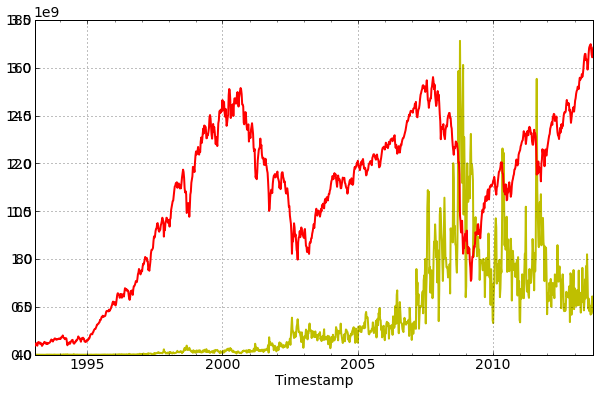## Regression analysis of S&P 500 data¶

In :
BB = history.OPEN.resample('1w', how='ohlc')
week_range = BB.high - BB.low
#week_range.describe()
week_return = BB.close / BB.open - 1
#week_return.describe()
MM = week_return.between_time('1993-01-31', '2013-09-15')
#lagged = MM.shift(1)
lagged = week_return.tshift(1, 'w').between_time('1993-01-31', '2013-09-15')
# Ordinary least squares
pd.ols(y=MM, x=lagged)

Out:
-------------------------Summary of Regression Analysis-------------------------

Formula: Y ~ <x> + <intercept>

Number of Observations:         1076
Number of Degrees of Freedom:   2

R-squared:         0.0012

Rmse:              0.0222

F-stat (1, 1074):     1.2743, p-value:     0.2592

Degrees of Freedom: model 1, resid 1074

-----------------------Summary of Estimated Coefficients------------------------
Variable       Coef    Std Err     t-stat    p-value    CI 2.5%   CI 97.5%
--------------------------------------------------------------------------------
x    -0.0344     0.0305      -1.13     0.2592    -0.0942     0.0254
intercept     0.0014     0.0007       2.07     0.0383     0.0001     0.0027
---------------------------------End of Summary---------------------------------


## And VWAP:¶

In :
MM = vwap / BB.open - 1
MM = MM.between_time('1993-01-31', '2013-09-15')
lagged = MM.tshift(1, 'w').between_time('1993-01-31', '2013-09-15')
pd.ols(y=MM, x=lagged)

Out:
-------------------------Summary of Regression Analysis-------------------------

Formula: Y ~ <x> + <intercept>

Number of Observations:         1076
Number of Degrees of Freedom:   2

R-squared:         0.0018

Rmse:              0.0122

F-stat (1, 1074):     1.9175, p-value:     0.1664

Degrees of Freedom: model 1, resid 1074

-----------------------Summary of Estimated Coefficients------------------------
Variable       Coef    Std Err     t-stat    p-value    CI 2.5%   CI 97.5%
--------------------------------------------------------------------------------
x    -0.0422     0.0305      -1.38     0.1664    -0.1020     0.0175
intercept     0.0003     0.0004       0.91     0.3610    -0.0004     0.0011
---------------------------------End of Summary---------------------------------


## Intro to Leveraged Finance: Decaying 3X Leveraged ETFs.¶

So the S&P did very well this year. Why can't we just invest into a 3x ETF and make 60 percent a year!

What are leveraged ETFs? First, ETFs (Exchange Traded Funds) and financial instruments that trade similarly to stocks, and attempt to replicate the return of a basket of other assets. In principle, it would require many costly transactions for an investor to replicate the exposure of an ETF, so they are seen as convenient cost saving tools (Example: one SPY purchase gives you the same exposure as buying every stock in the S&P 500). Some ETFs purport to replicate a multiple of the return of the underlying asset; these are known as Leveraged ETFs. Leveraged ETFs have an interesting behavior; many market participants have noted their long-term downward tendency. This is because they have an asymmetric distribution with a large chance of decay. While the chance of decay is large, the expected value of each timestep is the same as for a similar nonleveraged instrument. Let's attempt to demonstrate this through a Monte-Carlo approach.

### Use randn to simulate historical data¶

We want an etf security with normal historical returns. Average daily return should therefore be 0, with a standard deviation of 1%.

In :
# numpy/matplotlib
%pylab inline
history = randn(10000)
plot(randn(10000)) #plot the 10000-day historical price vector.
title('returns')
xlabel('day')
ylabel('return [%]')
remove_border()

Populating the interactive namespace from numpy and matplotlib

WARNING: pylab import has clobbered these variables: ['load', 'info', 'mpl', 'datetime', 'save', 'set_printoptions', 'unique']
%pylab --no-import-all prevents importing * from pylab and numpy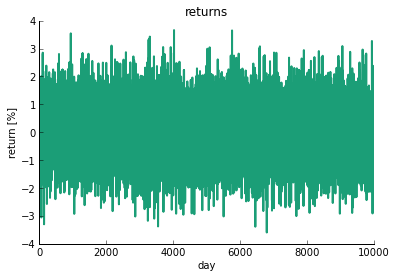In :
h = hist(history,100)
print 'Mean:' , history.mean()
print 'Standard deviation:%.2f' % history.std()

Mean: -0.00286332404091
Standard deviation:1.01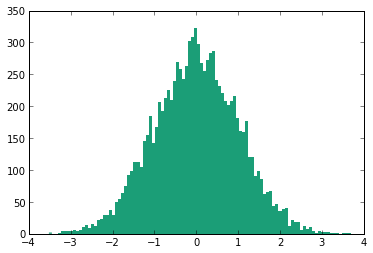This is very near mean 0, standard deviation 1.

Starting with an investmnt of k dollars, on day n our investment would then have a value of $k*r[t]*r[t+1]*...r[n]$. We can simulate these returns by using the cumulative product function.

In :
price = 100*(history/100+1).cumprod() # normalized price with a starting asset base of $100. plot(price) # cumulative sum title('normalized price') xlabel('day number') ylabel('price [$]')
remove_border()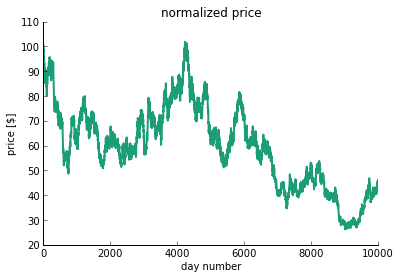Now employ a monte carlo approach, creating many simultaneous price paths.

In :
history = randn(1000,5000)
prices = 100*(history/100+1).cumprod(axis=0) # normalized price along axis
h = plot(prices[:,:300]) # plot first 300
xlabel('day number')
ylabel('price [$]') remove_border()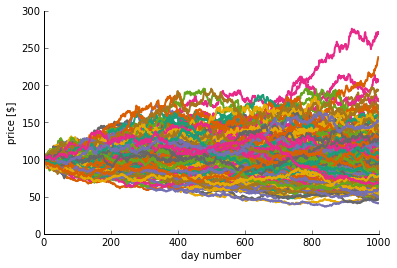Let's make a histogram of the last day of returns. This is the thousandth day, which is simply the end of the prices matrix. In : finalPrice = prices[-1,:] # get last row of prices table h = hist(finalPrice,50) # plot histogram xlabel('price [$]')
ylabel('frequency [#]')
print 'Mean:', finalPrice.mean()
print 'Std:', finalPrice.std()

Mean: 99.3375039092
Std: 32.1103764376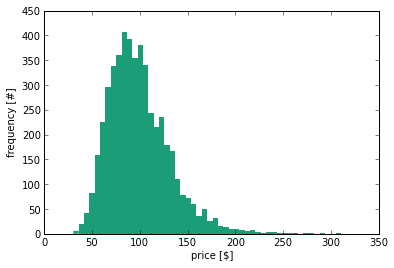## What about a leverage multiplier?¶

In :
history3x = history*3. # leveraged returns
prices3x = 100*(history3x/100+1).cumprod(axis=0) # normalized price
finalPrice3x = prices3x[-1,:] # last
h1 = hist(finalPrice3x,500) # histogram 1
h2 = hist(finalPrice,100) # histogram 2
xlim([0,400]) # setting limit of x axis
legend(['3 times','1 times'])
print 'Mean 3x:', finalPrice3x.mean()

Mean 3x: 98.0867193128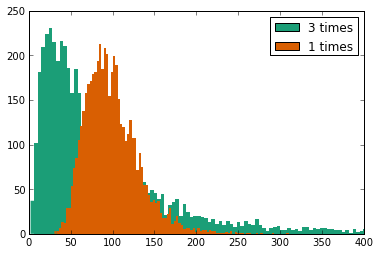The mean is close to our initial asset base, but the distribution is skewed to the right. No longer a normal distribution. Therefore, an equal probability of going up or down in percentage terms does not change long term expected value. The resulting distribution is however skewed involving a price decline more often, but it is compensated by the fat tails on the up side.

Note what is happening here: the fat tail of the leveraged ETF is so enormous that while the peak of the distribution is below 50, the long term mean is still at 100. This suggests that the naive strategy of shorting levereaged ETFs is not likely to be as profitable over the long term as it might at first appear.

So okay, our first approach did not work, but now to our next approach.

# Signal Processing ¶

In :
warnings.filterwarnings('ignore')
import signal_processing as sp
sp.example()

[2013-12-12 20:04] DEBUG: Transform: Running StatefulTransform [short_mavg]
[2013-12-12 20:04] DEBUG: Transform: Running StatefulTransform [long_mavg]
[2013-12-12 20:04] DEBUG: Transform: Finished StatefulTransform [long_mavg]
[2013-12-12 20:04] DEBUG: Transform: Finished StatefulTransform [short_mavg]
[2013-12-12 20:04] INFO: Performance: Simulated 253 trading days out of 253.
[2013-12-12 20:04] INFO: Performance: first open: 1990-01-02 14:31:00+00:00
[2013-12-12 20:04] INFO: Performance: last close: 1990-12-31 21:00:00+00:00

IBM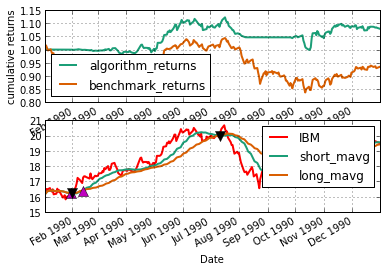### Dual Moving Average Cross (DMAC)¶

The concept of a DMAC is fairly straightforward. Calculate two moving averages of the price of a security, or in this case exchange rates of a currency. One average would be the short term (ST) (strictly relative to the other moving average) and the other long term (LT). Mathematically speaking, the long term moving average (LTMA) will have a lower variance and will move in the same direction as the short term moving average but at a different rate. The different rates of direction, induces points where the values of the two moving averages may equal and or cross one another. These points are called the crossover points.

In the dual moving average crossover trading strategy, these crossovers are points of decision to buy or sell the desired product. What these crossover points imply depends on the approach the investor has in their strategy. There are two schools of thought: Technical and Value.

The Technical Approach suggests that when the Short Term Moving Average (STMA) moves above the LTMA, that represents a Buy (or Long) signal. (Conversely, when the STMA moves below the LTMA, the Technical Approach indicates a Sell (or Short) signal.) The intuition behind this strategy can be explained in terms of momentum. Basically, the principle of momentum states that a price that is moving up (or down) during period t is likely to continue to move up (or down) in period t+1 unless evidence exists to the contrary. When the STMA moves above the LTMA, this provides a lagged indicator that the price is moving upward relative to the historical price. Buy high, sell higher.

The Value Approach offers the opposite trading signals to the Technical Approach. The Value Approach claims that when the STMA crosses from below to above the LTMA, that the product is now overvalued, and should be sold. Conversely when the currency STMA moves below the LTMA then the product is undervalued it should be bought. The intuition behind the Value Approach can be thought simply as a mean reversion approach. Buy low (value), sell high (overvalued).

Both strategies try to achieve the same goal, but do it in opposing ways to one another. In this paper, we will analyze both the technical and value strategies as applied to the SPY with the periods of 2 and 5.

Let us better understand what we are doing. We are looking for the underlying signal from a stock's price. We are then taking a value based approach and a technical approach using these signals. But what is a signal. Signals are tools for estimating future value of an asset based on its previous behavior, e.g. predict the price of AAPL stock based on its previous price movements for that hour, day or month. But what does it do? Consider below:

In :
# make a new dataframe from the original dataset
prices = pd.DataFrame(data.reindex( index=data.index[ ::-1 ] ).OPEN)
prices.plot()
remove_border()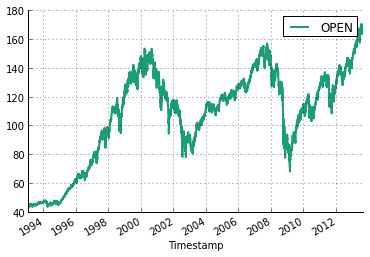Notice how choppy the graph appears. It is hard to tell which direction the S&P is moving. Now consider applying a simple moving average (see included python files: filters.py).

In :
prices['SMA50'] = sp.sma(prices.OPEN,50)
prices['SMA200'] = sp.sma(prices.OPEN,200)
prices.plot()
remove_border()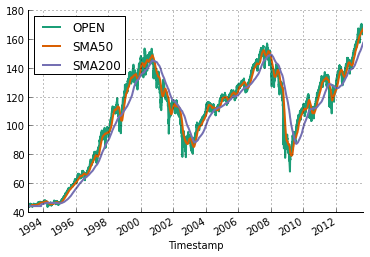Now what you see is a smoother set of curves. Notice how the 50 period SMA follows the actual prices more closely than the SMA 200 curve. And the 200 SMA follows it more loosely. Why? The 200 curve looks a prices up to 200 days ago, vs the 50 which only looks 50 days back. That is why the SMA 50 reacts more quickly.

Now that we understand what the true values/underlying signals of the market are. But how do we use this? Now we can implement a DMAC algorithm that based on these signals will produce equity curves (how much we will have made). Consider the code below.

In :
equity_curves = pd.DataFrame(sp.dmac(prices.OPEN,prices.OPEN,sp.sma,2, 5, 5, True),columns =['Value SMA'],index=prices.index)
equity_curves.plot(); remove_border()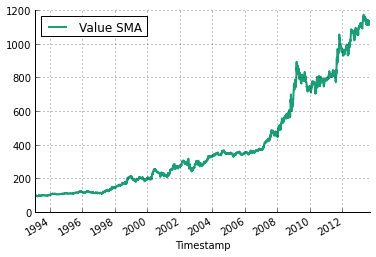Well hot dog! Looks like we have a winner. Well we should just go invest in this! It gives us 10x returns! Well wait, let's first put this in prespective

In :
equity_curves['Technical SMA'] = sp.dmac(prices.OPEN,prices.OPEN,sp.sma,2, 5, 5, False)
equity_curves['Baseline'] = 100*prices.OPEN/prices.OPEN

equity_curves.plot(); remove_border()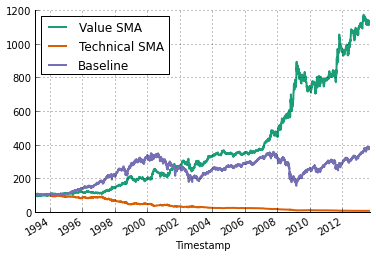Okay, this is more reasonalble. It seems that we have beaten the baseline in our Value base approach (described above) and lost nearly all of our money with our Technical appraoch. But generally speaking just eyeballing our results is not the best strategy, there are certain statistics that we should veiw:

## Statistics

Standard and Poor's (SPY) 500 Total Return Index is a capitalization-weighted index of 500 stocks. The index is designed to measure performance of the broad domestic economy through changes in the aggregate market value of 500 stocks representing all major industries. The index was developed with a base value of 10 for the 1941-1943 base period.

Annualized Standard Deviation is a statistical measure of the degree to which an individual value in a probability distribution tends to vary from the mean of the distribution. It is widely applied to Modern Portfolio Theory for example, where the past performance of securities is used to determine the range of possible future performances and a probability is attached to each performance. The standard deviation of performance can then be calculated for each security and for the portfolio as a whole. The greater the dispersion, the greater the risk.

Sharpe Ratio is the amount of reward per unit of risk. The higher the Sharpe Ratio, the more incremental return is added per increase in risk as measured by standard deviation.

Sortino Ratio is a ratio that differentiates between good and bad volatility in the Sharpe ratio. This differentiation of upwards and downwards volatility allows the calculation to provide a risk-adjusted measure of a security or fund's performance without penalizing it for upward price changes.

Alpha is a measure of excess return, or the amount by which a portfolio outperforms a benchmark, such as the T-bill rate. For example, an alpha of 1.20 indicates that a fund is expected to rise 20% during a given time period when the return on the market (and the fund's beta) are zero.

Beta is the measure of a fund's volatility relative to the market. (Many fund managers correlate themselves to the SPY 500.) A beta of greater than 1.0 indicates that the fund is more volatile than the market, and less than 1.0 is less volatile than the market. For example, if the market rises 1% and a fund has a beta equal to 2.5, then the fund is likely to rise faster than the market ( and conversely fall faster than the market when the market falls).

Correlation is the degree to which the movements of two variables are related. The correlation coefficient is expressed as a value between -1 and +1. For example,a correlation coefficient of 1 would suggest that two asset classes are perfectly correlated, a coefficient of zero suggests no relationship, and a coefficient of -1 suggests an inverse relationship.

R-squared is a statistical measure that represents the percentage of a fund or security's movements that can be explained by movements in a benchmark index.

Maximum Drawdown is the maximum peak-to-trough percentage decline in value experienced during the given period.

We will begin by examining the statistics on our baseline.

In :
stats = sp.performance(equity_curves['Baseline'], time_frame= 251)
pd.DataFrame([stats.values()],columns = stats.keys(),index=['Baseline'])

Out:
sharp std sortino car3 car1 cret pos car5 aret max ave ytd 1000
Baseline 0.4506 0.169088 0.868786 14.479322 17.134151 284.648624 0.526083 5.947035 6.967836 56.70044 0.033569 17.134151 3846.486241

So the baseline model (meaning let's just invest in the S&P 500) does pretty well. It gives us nearly 4x! But what else... The max drawdown was 54%. Pretty bad, during the morgage crisis the market really sank. The anuallized return was 7% a year! The sharp ratio (usually looked to be good at .5) was a bit above .45. But lets look at the statistics of all three combined.

In :
stats = []
for strategy in ['Baseline','Value SMA','Technical SMA']:
stats.append( sp.performance(equity_curves[strategy], time_frame= 251))

pd.DataFrame([stat.values() for stat in stats],columns = stats.keys(),index=['Baseline','Value SMA','Technical SMA'])

Out:
sharp std sortino car3 car1 cret pos car5 aret max ave ytd 1000
Baseline 0.450600 0.169088 0.868786 14.479322 17.134151 284.648624 0.526083 5.947035 6.967836 56.700440 0.033569 17.134151 3846.486241
Value SMA 0.857395 0.159222 16.518197 12.628268 4.673404 1021.574147 0.337055 14.389879 12.847359 24.042366 0.051072 4.673404 11215.741466
Technical SMA -1.141516 0.109234 -1.163120 -12.432710 -5.102461 -94.240220 0.307796 -15.226719 -13.299761 20.924733 -0.050313 -5.102461 57.597798

Now we can make some comparisons. First we notice that the Technical SMA did quite poorly as compared to the other strategies. In almost all regards. It lost money. Next is the Value SMA. It made more money. And in fact it is less risky, there is less volitility. We can see this by looking at the sharp and sortino ratios. But then two questions arise... Did we choose the correct period, and did we choose the correct filter. Let's deal with the second question first.

Let us deal with the first of these questions. We have done research, and the periods of 2 and 5 were recommended, but let us check a braoder range of periods.

In :
from mpl_toolkits.mplot3d import Axes3D

values = []
for short_period in range(1,5):
for long_period in range(short_period + 1, short_period + 5):
values.append([short_period, long_period,sp.dmac(prices.OPEN,prices.OPEN,sp.sma,short_period, long_period, 5, True,end_value=True)])

X,Y,Z = zip(*values)

print max(values,key=lambda x:x)

fig = plt.figure()
ax = Axes3D(fig)
ax.plot_trisurf(X,Y,Z)

[2, 5, 1121.5741465850183]

Out:
<mpl_toolkits.mplot3d.art3d.Poly3DCollection at 0x1632d8d0>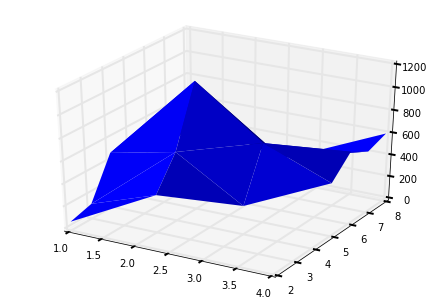So yes, our research worked out, in fact the 2 and 5 periods lead to the best overall gain. So the final question we will ask is: Is our filter right? We will focus on four different types of filters (all described and coded in signal_processing.py). The filters are

• SMA (simple moving average): This is the coarsest method of finding a trend. It weights past events the same as current events, which means it is relativly unresponsive
• EMA (exponential moving average): This uses exponential weighting. Thus more recent events are weighted more heavily.
• Gaussian Filter: This is a n order exponential moving average and is better able to capture price displacment because of events
• Butterworth Filter: More sophisticated infinite impulse response filter. It is a higher oder gaussian with two poles.

Let us then do a naive optimization over all of these filters to find their optimal periods, and then veiw some statistics.

In :
for filt,name in [(sp.ema,'EMA'),(sp.gaussian,'Gaussian'),(sp.butterworth,'Butterworth')]:
values = []
for short_period in range(1,5):
for long_period in range(short_period + 1, short_period + 5):
values.append([short_period, long_period,sp.dmac(prices.OPEN,prices.OPEN,filt,short_period, long_period, 5, True,end_value=True)])

optimal = max(values,key=lambda x:x)

print "%s Value, Returns: %d, Periods: %d, %d" % (name, optimal, optimal, optimal)

values = []
for short_period in range(1,5):
for long_period in range(short_period + 1, short_period + 5):
values.append([short_period, long_period,sp.dmac(prices.OPEN,prices.OPEN,filt,short_period, long_period, 5, False,end_value=True)])

optimal = max(values,key=lambda x:x)

print "%s Technical, Returns: %d, Periods: %d, %d" % (name, optimal, optimal, optimal)


EMA Value, Returns: 499, Periods: 1, 5
EMA Technical, Returns: 59, Periods: 4, 8
Gaussian Value, Returns: 457, Periods: 2, 3
Gaussian Technical, Returns: 42, Periods: 2, 6
Butterworth Value, Returns: 101, Periods: 1, 2
Butterworth Technical, Returns: 98, Periods: 1, 2


So we are done right? Not quite yet. So it may seem like SMA preforms the best out of all of the filters above. But we have not looked at their statistics. Let us veiw the best of the best, and compare SMA, EMA, and the baseline.

In :
    equity_curves['Value EMA'] = sp.dmac(prices.OPEN,prices.OPEN,sp.ema,1, 5, 5, True)
equity_curves['Value Gaussian'] = sp.dmac(prices.OPEN,prices.OPEN,sp.gaussian,2, 3, 5, True)
del equity_curves['Technical SMA']
equity_curves.plot()
remove_border()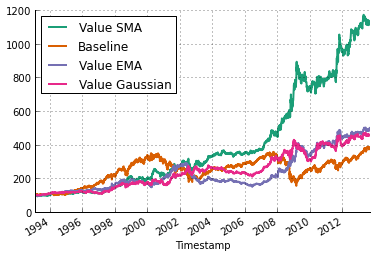Before we move on to the stats, let's take a look at what we have here. The most curious thing to note is that the EMA did not do too much better than the baseline. Oddly enough the EMA seemed to do best in times of economic downturn. Well, lets move onto the statistics, and comparative statistics.

In :
stats = []
for strategy in ['Baseline','Value SMA','Value EMA','Value Gaussian']:
stats.append( sp.performance(equity_curves[strategy], time_frame= 251))

pd.DataFrame([stat.values() for stat in stats],columns = stats.keys(),index=['Baseline','Value SMA','Value EMA','Value Gaussian'])

Out:
sharp std sortino car3 car1 cret pos car5 aret max ave ytd 1000
Baseline 0.450600 0.169088 0.868786 14.479322 17.134151 284.648624 0.526083 5.947035 6.967836 56.700440 0.033569 17.134151 3846.486241
Value SMA 0.857395 0.159222 16.518197 12.628268 4.673404 1021.574147 0.337055 14.389879 12.847359 24.042366 0.051072 4.673404 11215.741466
Value EMA 0.509205 0.185759 1.388655 8.647352 8.518586 399.564057 0.327045 11.128043 8.375112 48.362182 0.035617 8.518586 4995.640570
Value Gaussian 0.537531 0.168085 1.455399 4.046805 4.218977 357.432135 0.300866 6.198407 7.898730 30.130000 0.033532 4.218977 4574.321353
In :
stats = []
for strategy in ['Value SMA','Value EMA','Value Gaussian']:
stats.append( sp.stats(equity_curves[strategy], equity_curves['Baseline'],time_frame= 251))

pd.DataFrame([stat.values() for stat in stats],columns = stats.keys(),index=['Value SMA','Value EMA','Value Gaussian'])

Out:
alpha beta r return correlation
Value SMA 12.124887 0.021216 0.000268 1021.574147 0.016368
Value EMA 6.986131 0.019568 0.000234 399.564057 0.015300
Value Gaussian 6.260903 0.038330 0.000822 357.432135 0.028669

So this tells the story. The Value SMA has lower varience (meaning high sharp and sortino, and low maximum drawdown and standard deviation). And it has higher in terms of return. All of the strategies yeild positive alpha and have very low beta.

### DMAC Further Work¶

One can much more thruoghouly examine different filters and different periods in order to find the optimal. But we should be wary of overfitting. There are other statistical test that we could preform to judge the value of a strategy.

### RSI¶

RSI or relative strength index is a measure of a certain stock being overbought or oversold. Thus if the RSI (implemented and explained further in signal_processing.py) breaches upper and lower bounds of 70 and 30, we should assume that the stock will revert to the mean. But what does this look like?

In :
equity_curves = pd.DataFrame(sp.rsi(prices.OPEN,50),columns =['RSI'],index=prices.index)
equity_curves.plot(); remove_border()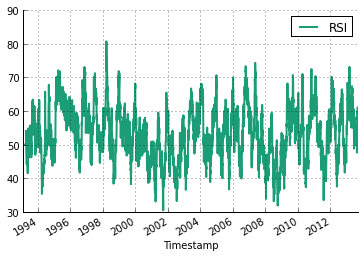Well this does not tell us much. First the principal of RSI is: Sell wehn above 70 and buy when below 30. So lets add some more graphics to the chart.

In :
equity_curves['Upper Bound'] = *len(equity_curves.RSI)
equity_curves['Lower Bound'] = *len(equity_curves.RSI)
# normalize the original s&p
equity_curves['Baseline'] = 100*prices.OPEN/prices.OPEN[-1]
equity_curves.plot(); remove_border(); plt.legend(loc='upper left')

Out:
<matplotlib.legend.Legend at 0x1be9fb00>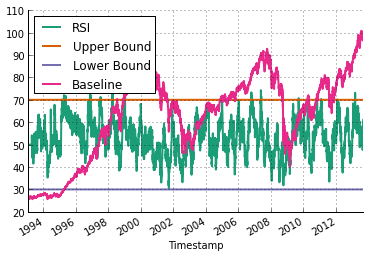Ah, now we can see the strength of RSI. Notice that when RSI breaks the bounds of 70/30, the morket will then move in the other direction. RSI tells us if the stock is overbought or oversold. Thus we can see that it works, to an extent. Let's try to find the best implementation of this strategy.

In :
values = []
for period in range(1,101):
values.append([period,sp.equity_curve(sp.rsi(prices.OPEN,period), prices.OPEN, *len(prices.OPEN), *len(prices.OPEN), 5,end_value=True)])

print max(values,key=lambda x:x)

periods, returns = zip(*values)

plt.plot(returns)

[13, 114.08440469332842]

Out:
[<matplotlib.lines.Line2D at 0x1babc518>]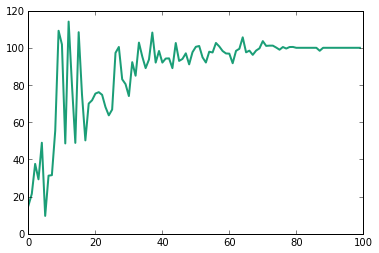So as one can see, there is a trend to RSI such that by increasing or decreasing the period can lead to consistantly better results. Notice that as the period increases, we get less volitility, because we get fewer trades. But we have found a generally optimal strategy. Let us view some statistics on this curve vs. the baseline and finish off with our conclusions on RSI.

In :
equity_curves = pd.DataFrame(sp.equity_curve(sp.rsi(prices.OPEN,13), prices.OPEN, *len(prices.OPEN), *len(prices.OPEN), 5),columns =['RSI'],index=prices.index)
# normalize the original s&p
equity_curves['Baseline'] = 100*prices.OPEN/prices.OPEN
equity_curves.plot(); remove_border();# plt.legend(loc='upper left')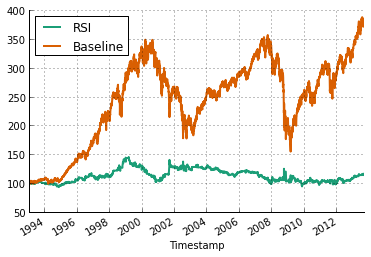So RSI might not be what we are looking for. RSI is a naive approach to handle the stock market from the 1970s and it is not surprising that such a naive strategy might not work. Further implemntations might be to change the holding period, to set stops, or in short develop a more comprehensive RSI tool.

### Conclusions¶

Signal processing can get us far. It can make us money, ensure low volitility, and even beat the stock market. But sometimes it just does not work. Our naive approach to signal processing has given us insight on how traders might look at the market and how to evaluate a strategy, however there exist problems with overfitting and a potentially limitless supply of different signals.

Extensions and future work for signal processing include: run more advanced statistics, to try a wider range of techniques, and develop a more in depth trading system (including stops, limits, commissions, and slippage).

# Portfolio Construction and Analysis¶

This is the most classice way to go about making money on the market: buying some stocks and putting them in your portfolio.

## Calculate returns from price data.¶

Returns defined as:  $$r_t = \frac{p_t-p_{t-1}}{p_{t-1}}$$

We define the iterative return index:

$$i_t = (1 + r_t) \cdot i_{t-1}, \quad i_0 = 1$$
In :
RR = close_px/close_px.shift(1) - 1
daily_index = (1 + RR).cumprod()
daily_index['MSFT'].plot()
remove_border()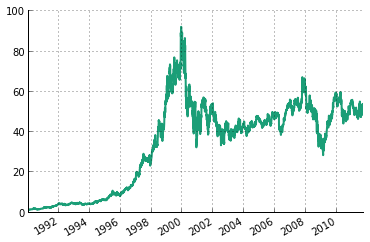In :
# Let's sample and plot monthly returns...
monthly_index = daily_index.asfreq('EOM', method='ffill')
monthly_RR = monthly_index / monthly_index.shift(1) - 1
monthly_RR.plot()
remove_border()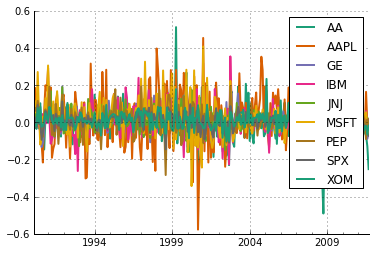We can plot multiple timeseries in a dataframe using matplotlib:

In :
subRR = RR.ix[:, ['AAPL', 'IBM', 'XOM', 'MSFT']]
(1 + subRR).cumprod().plot()
remove_border()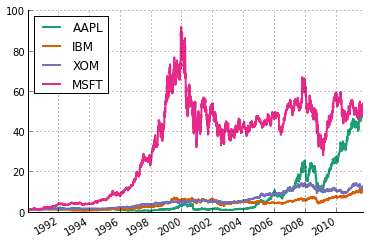We can look at the distributions of stock and ETF (Exchange Traded Fund) returns:

In :
RR[-250:].hist(bins=50)
remove_border()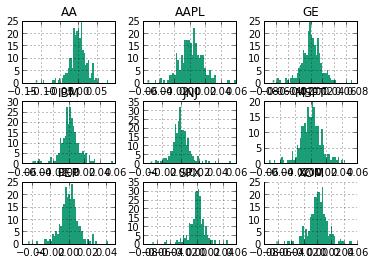In :
RR.ix[-1].plot(kind='bar')
remove_border()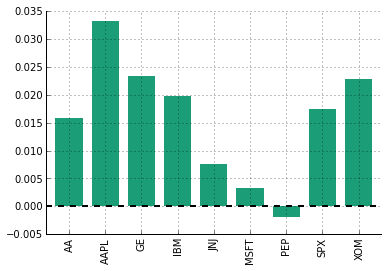We can use a sliding window of price to calculate certain functions of timeseries, such as rolling volatility and moving averages.

In :
# We have the following plot for Apple:
px = close_px['AAPL']
close_px['AAPL'].plot()
remove_border()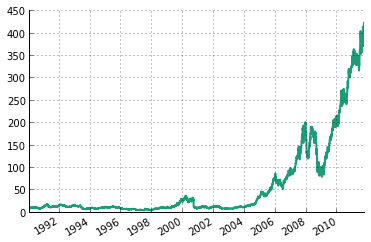And the corresponding rolling volatility:

In :
vol = rolling_std(px / px.shift(1) - 1, 250) * np.sqrt(250)
vol.plot()
remove_border()